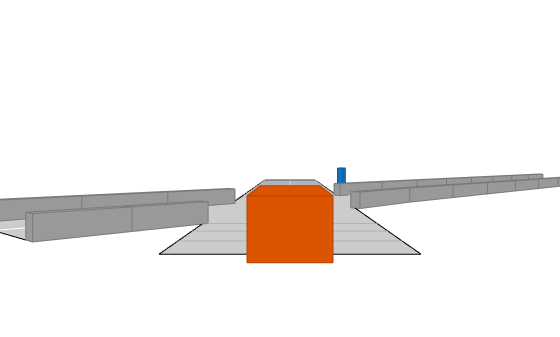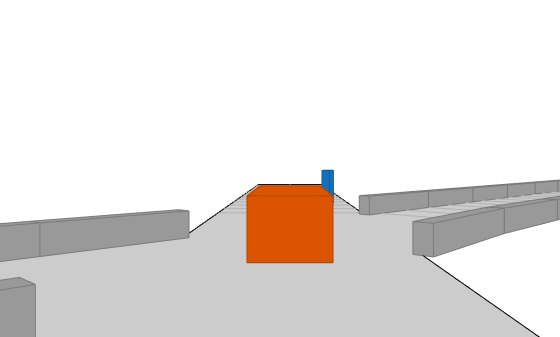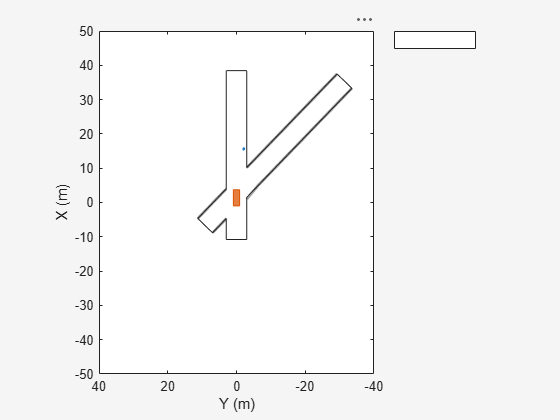Main Content

# targetOutlines

Outlines of targets viewed by actor

## Syntax

``[position,yaw,length,width,originOffset,color] = targetOutlines(ac)``
``[position,yaw,length,width,originOffset,color,numBarrierSegments] = targetOutlines(ac,'Barriers')``

## Description

example

````[position,yaw,length,width,originOffset,color] = targetOutlines(ac)` returns the oriented rectangular outlines of all non-ego and non-barrier target actors in a driving scenario. The outlines are as viewed from a designated ego vehicle actor, `ac`. See Ego Vehicle and Targets for more details.A target outline is the projection of the target actor cuboid into the (x,y) plane of the local coordinate system of the ego vehicle. The target outline components are the `position`, `yaw`, `length`, `width`, `originOffset`, and `color` output arguments.You can use the returned outlines as input arguments to the outline plotter of a `birdsEyePlot`. First, call the `outlinePlotter` function to create the plotter object. Then, use the `plotOutline` function to plot the outlines of all the actors in a bird's-eye plot.```
````[position,yaw,length,width,originOffset,color,numBarrierSegments] = targetOutlines(ac,'Barriers')` returns only the oriented rectangular outlines of all barriers in a driving scenario. The additional output argument `numBarrierSegments` contains the number of segments present in each barrier.You can use the retuned barrier outlines as input arguments to the outline plotter of a `birdsEyePlot`. First, call the `outlinePlotter` function to create the plotter object. Then, use the `plotBarrierOutline` function to plot the outlines of all the barriers in a bird's-eye plot. ```

## Examples

collapse all

Create a driving scenario and show how target outlines change as the simulation advances.

Create a driving scenario consisting of two intersecting straight roads. The first road segment is 45 meters long. The second straight road is 32 meters long with jersey barriers along both its edges, and intersects the first road. A car traveling at 12.0 meters per second along the first road approaches a running pedestrian crossing the intersection at 2.0 meters per second.

```scenario = drivingScenario('SampleTime',0.1,'StopTime',1); road1 = road(scenario,[-10 0 0; 45 -20 0]); road2 = road(scenario,[-10 -10 0; 35 10 0]); barrier(scenario,road1) barrier(scenario,road1,'RoadEdge','left') ped = actor(scenario,'ClassID',4,'Length',0.4,'Width',0.6,'Height',1.7); car = vehicle(scenario,'ClassID',1); pedspeed = 2.0; carspeed = 12.0; smoothTrajectory(ped,[15 -3 0; 15 3 0],pedspeed); smoothTrajectory(car,[-10 -10 0; 35 10 0],carspeed);```

Create an ego-centric chase plot for the vehicle.

`chasePlot(car,'Centerline','on')`Create an empty bird's-eye plot and add an outline plotter and lane boundary plotter. Then, run the simulation. At each simulation step:

• Update the chase plot to display the road boundaries and target outlines.

• Update the bird's-eye plot to display the updated road boundaries and target outlines. The plot perspective is always with respect to the ego vehicle.

```bepPlot = birdsEyePlot('XLim',[-50 50],'YLim',[-40 40]); outlineplotter = outlinePlotter(bepPlot); laneplotter = laneBoundaryPlotter(bepPlot); legend('off') while advance(scenario) rb = roadBoundaries(car); [position,yaw,length,width,originOffset,color] = targetOutlines(car); [bposition,byaw,blength,bwidth,boriginOffset,bcolor,barrierSegments] = targetOutlines(car,'Barriers'); plotLaneBoundary(laneplotter,rb) plotOutline(outlineplotter,position,yaw,length,width, ... 'OriginOffset',originOffset,'Color',color) plotBarrierOutline(outlineplotter,barrierSegments,bposition,byaw,blength,bwidth, ... 'OriginOffset',boriginOffset,'Color',bcolor) pause(0.01) end```## Input Arguments

collapse all

Actor belonging to a `drivingScenario` object, specified as an `Actor` or `Vehicle` object. To create these objects, use the `actor` and `vehicle` functions, respectively.

## Output Arguments

collapse all

Rotational centers of targets, returned as a real-valued N-by-2 matrix. N is the number of targets. Each row contains the x- and y-coordinates of the rotational center of a target. Units are in meters.

Yaw angles of targets about the rotational center, returned as a real-valued N-element vector. N is the number of targets. Yaw angles are measured in the counterclockwise direction, as seen from above. Units are in degrees.

Lengths of rectangular outlines of targets, returned as a positive, real-valued N-element vector. N is the number of targets. Units are in meters.

Widths of rectangular outline of targets, returned as a positive, real-valued N-element vector. N is the number of targets. Units are in meters.

Offset of the rotational centers of targets from their geometric centers, returned as a real-valued N-by-2 matrix. N is the number of targets. Each row contains the x- and y-coordinates defining this offset. In vehicle targets, the rotational center, or origin, is located on the ground, directly beneath the center of the rear axle. Units are in meters.

RGB representation of target colors, returned as a nonnegative, real-valued N-by-3 matrix. N is the number of target actors.

Number of barrier segments in each barrier, returned as a nonnegative, real-valued N-by-1 vector. N is the number of barriers in the scenario. This argument is returned only when the `'Barriers'` flag is provided as input.

## More About

collapse all

### Ego Vehicle and Targets

In a driving scenario, you can specify one actor as the observer of all other actors, similar to how the driver of a car observes all other cars. The observer actor is called the ego actor or, more specifically, the ego vehicle. From the perspective of the ego vehicle, all other actors (such as vehicles and pedestrians) are the observed actors, called targets. Ego vehicle coordinates are centered and oriented with reference to the ego vehicle. The coordinates of the driving scenario are world coordinates.

Introduced in R2017a

## Support

#### Implementing an Adaptive Cruise Controller with Simulink

Download technical paper